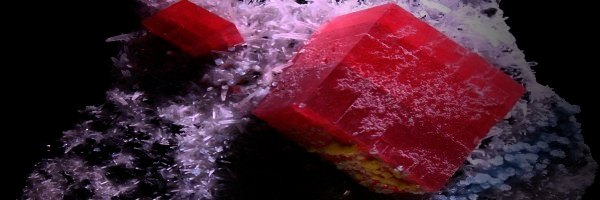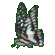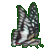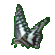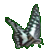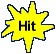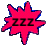Volume (Capacity) of Rectangular Solids & Cylinders & Quadrangular Prisms    Game Tips:

- In this game, you can find Volume (or capacity) by multiplying base Area times Height. Use V=AH.
The diagrams shown are not drawn to scale size.

- If the object has a rectangular base, then the base Area equals Length times Width. Use A=LW.
- You can use V=AH for the volume of rectangular solids and quadrangular prisms.

- If the object has a circular base, the base Area equals (Pi) times Radius times Radius.
Use the circular area formula A=(Pi)(R2), where the value of 'Pi' or 'π' is approximately '3.14' .

- You can use V = πR2H for the volume of a cylinder.

- Your Score Report appears after you have made 8 choices.
- Your Game Score is reduced by the number of butterfly hits.

- To slow the game speed repeat tap/click on the word Slider.
- To increase the game speed repeat tap/click on the word Math.
- Speed can also be adjusted with a keyboard's - and + keys.

- Refresh/Reload the web page to restart the game.
- Adjust the sound level of media on your device.

More SliderMath